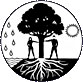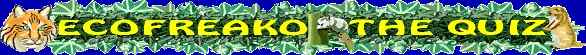(Easy Quiz) There are five easy level questions. Your maximum score is 100. All hints are free. Each correct answer gives a score of 20. (Medium Quiz) There are five medium level questions. Your maximum score is 100. Hints cost. If you do not use your hint then each correct answer gives a score of 20 and if you get it wrong you will score 0 for that question. If you use a hint to answer the question you will only get only 50% of the points for that question if you get it right and so will score 10. But if you get it wrong you will lose 10. (Hard Quiz) There are ten medium to hard level questions. You score 10 for each correct question answered. Your maximum score is 100. No hints are available.